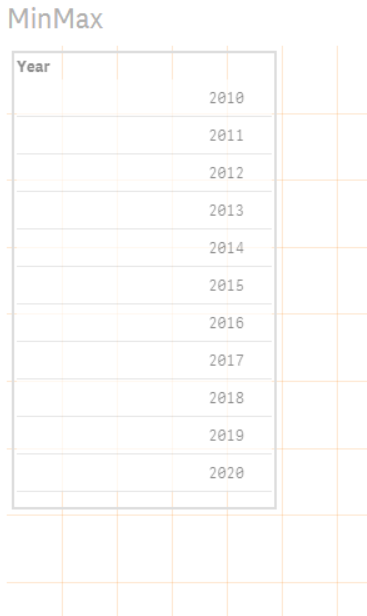# Qlik Sense Documents & Videos

Documents & videos about Qlik Sense.

Announcements
See why Qlik is recognized as a Leader for the 10th year in a row – and discover how Qlik can help put your business in the lead. Get Report

# Howto - Get minimum and maximum value of a selectionPartner

## Howto - Get minimum and maximum value of a selection

I was wondering if it was possible to determine / calculate the minimum and maximum value of a selected field (in a filter pane) and with a few hours of investigation I succeeded.

For this example I used a new app and added the following data load:

Years:

[

Year

2010

2011

2012

2013

2014

2015

2016

2017

2018

2019

2020

];

2. Load the data and insert a filter pane

Next step is to load the data and insert a new filter pane. Add field Year as a filter dimension:3. Insert a table and add a formula on dimension for the minimum selected year

First insert a table and add the formula:

=SubField(GetFieldSelections([Year],',',GetSelectedCount([Year])),',',1)

Explanation:

The formula GetFieldSelections() results in a string with the selected values of a field.

Field

The field within a filter pane, in this example field [Year]

Value_sep

The seperator to use between the selected values, in this example a comma (',')

Max_values

The maximum number of values that may be added in the string. To add all the selected values to the string, you can use GetSelectedCount() formula. This is the number of selected values of a field. If you select one year, the result of the formula GetSelectedCount([Year]) is 1, if you select two years, the result will be 2, if you select all years, the result will be 10.

The next formula that is needed to determine the minimum value of all the selected values is SubField(). This is a formula to get one value in a string of values seperated by a specific seperator.

Text expression

The text expression for this example is a string with all selected values: GetFieldSelections([Year],',',GetSelectedCount([Year]))

Delimiter

Use the same delimiter as the value_seperator, in this example use comma (',')

Field_no

To retrieve the first selected value, use number 1.

4. Add a formula on dimension for the maximum selected year

Add the following formula to determine the maximum value of the selected year:

=SubField(GetFieldSelections([Year],',',GetSelectedCount([Year])),',',GetSelectedCount([Year]))

Explanation:

The same as the formula for minimum value, but instead of number 1, you have to retrieve the maximum number and that is: GetSelectedCount([Year])

5. Add a formula to determine if current year is in the selection

The previous formula can be combined with the formula SubStringCount() and then you can determine if the current year is part of the selection:

=If(SubStringCount(GetFieldSelections([Year],',',GetSelectedCount([Year])),Text(Year(Today()))), 'Yes', 'No')

Explanation:

With the formula SubStringCount(Text expression, sub_string) you can search how many times the sub_string occurs in the Text expression.

In this example: how many times occurs Text(Year(Today())) in GetFieldSelections([Year],',',GetSelectedCount([Year])).

In 'normal' language: how many times occurs Current year (converted to text) in String with all the selected values of field Year.I hope this make sense and you can use this...Not applicable

Thank you for sharing..Not applicable

Very usefull, thank you !

Version history
Revision #:
1 of 1
Last update:
‎2015-07-28 11:51 AM
Updated by: